Graphing Basic Trig Functions

 Graph y = sin(x)

Enter the function.              Set the MODE to Radian.        Choose ZOOM #7 ZTrig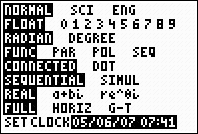Choosing ZOOM #7 ZTrig will set the x interval from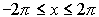,
set the y interval from -4 < y < 4, and set the increment at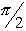.
Depending upon your desired graph, you may wish to further adjust these values under
WINDOW.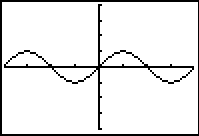NOTE:  If you do a ZOOM #7 ZTrig while in DEGREE mode, the x interval will be set from -352.5ş to 352.5ş, with the y interval from -4 to 4, and the increment set at 90ş.

 Graph y = tan(x)

Enter the function.              Set the MODE to Radian.        Choose ZOOM #7 ZTrig"Wow!  Look!  It even graphed the asymptotes!!" N O T !!!!!!!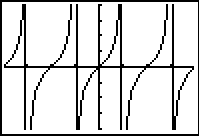NOTE:  Starting with the TI-84+ Operating System V 2.40, this problem no longer exists.

These vertical lines are not the asymptotes.  They are simply the calculator behaving as it should in "Connected" mode -- it is "connecting" the points.
 Here is the graph in DOT mode.  No asymptotes!!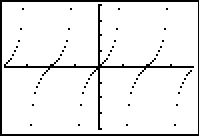Graph  y = 2sin(3(x - 2)) + 12

This is a sinusoidal equation of the form y = Asin(B(x - C)) + D, where A is the amplitude, B is the frequency, C is the horizontal shift and D is the vertical shift.  The period of this graph will beWhen graphing such equations, it may be necessary to adjust the window to "see" the graph.  If ZOOM #7 Trig is used with this graph, the screen will appear empty.  The vertical shift tells you that the graph has been "raised" by 12 units.  Adjust the yMax and yMin accordingly.  The yMin has been set to 0 for this example to establish a reference point when viewing the graph.

Enter the function.              Set the MODE to Radian.       Adjust the ZOOM #7 ZTrig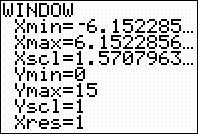Graph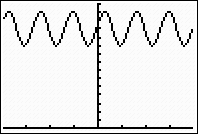Adjust the WINDOW further for additional examinations of the graph.